# Authors

 Ephraim M. Sparrow Edward G. Palmer John A. Sipple SolarAttic Department of Mechanical Engineering Minnesota University of Minnesota Minneapolis, MN 55455

First Published
Solar 95 Conference Proceedings
American Solar Energy Society
2400 Central Avenue, Suite G-1
Boulder, CO 80301

ABSTRACT

A novel solar concept is the utilization of existing attic spaces as solar collectors. A heat
exchanger situated in the attic facilitates the utilization of the solar-heated attic air to create
useful energy products such as heated swimming-pool water and residential hot water. To
enhance these products, a method is developed here to increase the energy carried into the heat
exchanger by the solar-heated air. The basic idea is to utilize all parts of the attic as a hot-air
reservoir rather than only the immediate neighborhood of the heat exchanger inlet face. In the
practical realization of this idea, a flexible conduit attached to the heat exchanger inlet is
deployed throughout the attic. The wall of the conduit is made permeable to enable the ingestion
of air into the conduit from all neighborhoods along its length. The far end of the conduit is
capped. An analytical model is developed which yields a specification of the axial distribution
of the permeability needed to achieve axially uniform air ingestion. An apparatus was built to
validate the model and its predictions. The measured axial pressure distributions were in very
good agreement with that predicted from the analysis. This agreement validates the model and
supports its further use as a design tool for enhancing the utilization of attic-collected solar
energy. Key Words: solar energy, attic-collected solar, swimming-pool water heating, residential
water heating.

#### 1. INTRODUCTION

There are numerous approaches for collecting and utilizing solar energy for residential

purposes (1,2). These approaches can be classified as either active or passive. Active solar is

characterized by the use of dedicated equipment for collecting the incident flux of solar energy.

On the other hand, passive solar utilizes the physical structure of the residence to perform the

collection function.

The concept to be dealt with here is based on using existing attic spaces as solar

collectors. In that sense, it is definitely a passive approach. However, some of the modes of

utilizing the attic-captured solar radiation are similar to those for active systems. For instance,

the attic-collected energy can be used for heating swimming pool water and even for heating

residential hot water.

The analysis of attic-based collection systems naturally subdivides itself into two parts.

One part is concerned with the techniques and equipment (if any) by which the actual energy

collection is accomplished. The other part has to do with the modalities and equipment for

utilizing the collected solar energy. In this paper, attention will be focused on the collection

function.

It is, of course, a common experience to encounter elevated temperatures in an attic space

on whose roof or side walls solar energy is incident. Attic temperatures of 120°F (49°C) are

commonplace and temperatures of 150°F (65°C) are possible. The temperature level that is

attained depends on the intensity of the insolation, the presence or absence of insulation and of a

radiation barrier at the interior surface of the roof, and the strength of the wind-based, forced

convection heat transfer at the exterior surface of the roof. It is to be expected that the

temperature will not be uniform throughout the air that fills the attic space. The degree of non

uniformity will be influenced by the degree of venting, the geometry of the attic, and the quality

of the insulation at the floor of the attic.

2 of 18

At the heart of present systems for utilizing attic-collected solar energy (3) is an air-to-

liquid (typically water) heat exchanger. In principle, the exchanger should be positioned in that

part of the attic where the highest air temperatures occur. However, in practice, the positioning

is often governed by geometric issues such as where the attic height is sufficient to accommodate

the height of the exchanger. As a consequence, there is a possibility that the exchanger may not

be situated optimally from the standpoint of energy utilization.

In connection with efficient energy utilization, there is an issue even more basic than the

placement of the heat exchanger. That issue is the possible short circuiting of the air discharged

from the heat exchanger directly back into the inlet face of the exchanger. Such a flow pattern is

illustrated schematically in Fig. 1. As seen there, the discharge flow may loop around to the inlet

without intervening contact with the hot surfaces of the attic, i.e., the roof and, perhaps, the side

walls. Such contact is essential to efficient use of the attic-collected solar energy. Indeed, in the

ideal manifestation of the utilization of such energy, the air discharged from the heat exchanger

would experience numerous contacts with the hot walls before it is returned to the inlet face of

the exchanger.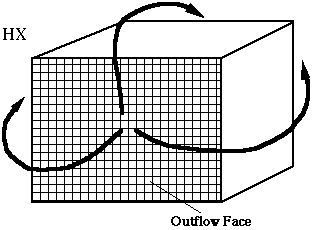Fig. 1 Representation of Flow Short-circuiting

3 of 18

The foregoing discussion sets the stage for the subject matter of this paper. The objective

of the paper is to propose and substantiate a method for creating a pattern of fluid flow within the

attic space which maximizes the utilization of the attic-collected solar energy.

#### 2. FLOW CONTROL APPROACH

A clear guideline for the attainment of extensive contact between the attic airflow and its

bounding walls is to establish a large separation distance between the inflow and outflow faces

of the heat exchanger. This can be accomplished in practice by ducting the air via a conduit from

the outflow face of the heat exchanger to some location remote from the inflow face, and

discharging it there. The thus-discharged air would then find its way to the inlet on its own. An

alternative would be to attach one end of a conduit to the inflow face of the exchanger and to

position the other end at a location remote from both the inflow face and the outflow face. This

remote positioning would force the air that is discharged at the outflow face to traverse a

considerable length of the attic before it reaches the open end of the conduit, from where it would

be ducted directly to the inlet.

Both of these arrangements have a flaw which is conveniently discussed with reference to

the latter of the two setups. In that case, the heat exchanger would be fed by air that is drawn

only from the immediate neighborhood of the open end of the conduit. No air would be taken

from the many neighborhoods situated adjacent to the cylindrical wall of the conduit. The

proposed remedy is to make the conduit wall permeable by creating a set of discrete holes in the

material from which the wall is made.

This is the concept that will be developed here. It will be developed in accordance with

the intuitive perception that the greatest flux of energy carried into the heat exchanger will be

achieved when the air is ingested uniformly into the permeable-walled conduit at all locations

along the length of the conduit.

4 of 18

An analysis will now be made to determine the distribution of the holes in the conduit

wall which provides the desired uniform ingestion. Subsequently, experiments performed to

verify the results of the analysis will be described.

#### 3. ANALYSIS

The fluid flow in the permeable-walled conduit will be analyzed by making use of the

equations for mass and momentum conservation. To facilitate the analysis, attention may be

directed to Fig. 2. Figure 2 is a schematic side view of the permeable-walled conduit. As seen

there, the conduit has a diameter D and length L. The axial coordinate in the flow direction is x,

where x = 0 corresponds to the end of the conduit that is farthest from the heat exchanger and

x = L denotes the end of the conduit that interfaces with the inlet of the heat exchanger. The

diagram also indicates that the far end of the conduit is capped. This practice was adopted

because an open-ended conduit would necessarily ingest much more air at its open end than

from other neighborhoods that are adjacent to the conduit wall. This situation would not be

compatible with the aforementioned goal of uniform ingestion.

###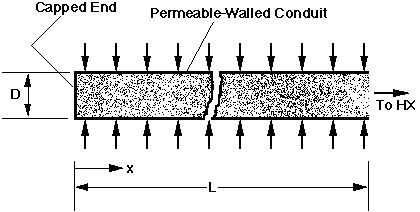Fig. 2 Schematic Diagram of the Permeable-Walled Conduit

5 of 18

At any cross section x, the mass flow rate of air is m(x). The rate at which air passes into

the conduit through its permeable wall in a length dx is dm, subject to the constraint

dm/dx = constant = M (1)

Since the x = 0 cross section is capped, m(0) = 0. It then follows from equation (1) that

m(x) = [x/L]m(L) (2)

where m(L) is the rate of mass flow at the inlet of the heat exchanger (i.e., at the exit of the

conduit). In the subsequent numerical evaluation of the analytical results, m(L) will serve as a

prescribable parameter (in actuality, the volumetric counterpart of m(x) will be prescribed).

The air that permeates through the wall of the conduit is driven by a pressure difference.

Let p(amb) be the pressure in the attic space adjacent to the conduit and p(x) be the pressure in

the conduit at a cross section x. Then, the local driving force for permeation is p(amb) - p(x).

This pressure drop results from two processes: (a) the acceleration experienced by the air as it

passes from the relatively quiescent attic ambient to the outer surface of the permeable-walled

duct, and (b) the flow resistance of the permeable wall. These components are, respectively, one

velocity head = 0.5 p[V(wall,x)]2 (3)

in which V(wall,x) is the velocity of the air that is moving radially inward through the permeable

wall at location x. Therefore,

p(amb) - p(x) = 1.5 velocity heads (4)

and from equations (3) and (4)

###(5)

6 of 18

The next step is to write an expression for the ingestion rate dm. For an axial length dx,

the surface area of the conduit is pDdx. A fraction of this area b(x) is open to permit ambient air

to pass into the conduit, where b is recognized to be a function of x. Therefore, in the length dx,

### d(free flow area) = b(x)pDdx

(6)

It follows that the rate of ingestion dm in an axial length dx is

dm = p[V(wall,x)]b(x)pDdx (7)

Then, with equation (5),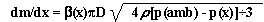(8)

The desired design condition is that dm/dx = constant = M (equation (1). Equation (8) can then

be solved for b (x)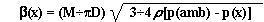(9)

Equation (9) is a means for obtaining numerical values for the free flow area factor b, provided

that the axial pressure distribution p(x) is known. The next part of the analysis deals with p(x).

The momentum conservation principle is the basis for the derivation of p(x). The

derivation is facilitated by reference to Fig. 3, which shows a control volume ( CV) and

nomenclature. Conservation of momentum may be written in any coordinate direction. For

present purposes, the appropriate direction is the x-direction. The momentum conservation

principle states that the difference between the rate of x-momentum that is carried out of a

control volume and the rate of x-momentum that is carried into the control volume has to equal

the net x-direction force. Figure 3 identifies the outflowing and inflowing x-moments and the x-

direction stresses.

7 of 18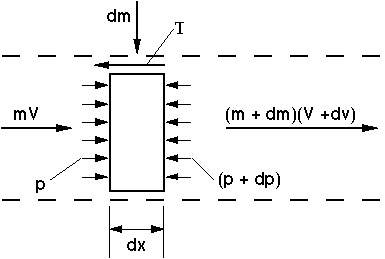Fig. 3 Control Volume

The velocity V denotes the cross-sectional average velocity. The pressures p and (p + dp) act

on the cross-sectional area of the control volume, while the shear stress T acts on the cylindrical

area that forms the interface between the control volume and the conduit wall. The areas

are, respectively,

cross-sectional area = ÆD2 Æ 4

(10)

area of CV-wall interface = ÆDdx

(11)

With these, the mathematical representation of the x-momentum balance can be written as

### (m + dm) (V +dV) - mV = {[p - (p + dp)]ÆD2 Æ 4} - TÆDdx

(12)

8 of 18

At any cross section,

m(x) = rV(x)ÆD2Æ4

(13)

and

dV = dmÆ[rÆD2Æ4]

(14)

When equations (13) and (14) are introduced into the momentum balance (12), there follows,

-dp/dx = 2m[dm/dx]Ær[ÆD2Æ4]2 + 4TÆD

(15)

Equation (15) is much simplified when it is recognized that dm/dx = constant = M. To

proceed, the shear stress appearing in the last term of equation (15) may be eliminated by

introducing the friction factor f via the definition

4T = [rV2Æ2]f

(16)

or, upon elimination of V by means of equation (13),

### 4T = {m2Æ2r[ÆD2Æ4]2}f

(17)

The friction factor f is a function of the Reynolds number Re of the air flowing in the conduit.

There are many available algebraic relationships between the friction factor and the Reynolds

number. The well-established Blasius formula will be used here because it enables a closed-

form, analytical, algebraic solution to be obtained for p(x). The Blasius formula can be written

as

### f = 0.316 Æ Re0.25

(18)

9 of 18

and the Reynolds number is

Re = 4m Æ µÆD

(19)

After substitution of equations (17), (18) and (19) and the introduction of dimensionless

variables, the governing equation (15) for pressure distribution emerges in a remarkably simple

form:

(20)

where

### X = x/L, Re(L) = 4m(L) Æ µÆD

(21)

and

P(X) = [p(amb) - p(x)] Æ 0.5r[V(L)]2

(22)

V(L) = m(L) Æ r[ÆD2Æ4]

(23)

Equation (20) is easily integrated to give P(x). Since it is a first-order differential

equation, one boundary condition must be provided. For the contemplated design methodology,

the state of the air flowing into the heat exchanger would be specified. This includes the desired

volumetric flow rate in cubic feet per minute (which is equivalent to V(L)) and the allowable

pressure drop [p(amb) - p(L)]. With these, the value of P(1) can be specified, and this serves

as the boundary condition for the differential equation (20).

The solution for the pressure distribution which incorporates the known boundary

condition is

### P(X) - P(1) = 2[X2-1] + 0.3636[LÆD] [Re(L)]-0.25[X2.75-1]

(24)

10 of 18

To extract a more physical form of equation (24), use may be used of the identity

[p(x) - p(L)]/[p(amb) - p(L)] = [P(X) - P(1)]/P(1)

(25)

Equation (25) enables the pressure distribution along the conduit to be presented in

dimensionless form as a function of x/L.  For the determination of the free flow area factor b(x),

which is needed for the specification of the holes in the surface of the conduit, the quantity

[p(amb) - p(x)]
is required as input to equation (9). To extract this pressure difference from

equation (24), it may be noted that

[p(amb) - p(x)] = [P(X)/P(1)] [p(amb) - p(L)]

(26)

where [p(amb) - p(L)] is a specified design parameter.

#### 4. RESULTS AND COMPARISONS WITH EXPERIMENTAL DATA

The simplicity of the algebraic equations which convey the results precludes the need for

an exhaustive graphical presentation. Such a presentation would be mandatory had a numerical

solution (e.g., finite-difference or finite-element) been required. In view of this, the graphical

presentation of results will be confined to the operating conditions of a complementary

experimental study which will now be described.

A 25-foot-long (7.6 m), 10-inch diameter (0.25 m), commercially available conduit was

used for the experiments. It consisted of a tightly stretched, impermeable plastic skin firmly

supported by an embedded helical wire. In its as-arrived state, the conduit was open at both

ends. The blower procured for the experiments was situated in a rectangular housing, the inlet

face of which was a 12.5-inch-diameter (0.32 m) circle, and the exit face of which was a 14-inch

(0.36 m) square. The blower was driven by a 1/12th hp electric motor. A conical transition

piece was fabricated to facilitate the mating of one end of the conduit with the inlet face of the

blower.

11 of 18

Two quantities were measured in order to test the analytical predictions: (a) volumetric

air flow rate and (b) the pressure distribution p(x)along the length of the conduit. The

volumetric flow rate was determined by a velocity traverse across the fully exposed exit face of

the blower housing. For this purpose, the exit face was subdivided into a 7 by 7 grid to define 49

equally deployed measurement sites. The local velocities on this grid were measured with a hot-

film anemometer whose electronics provided a digital readout which was manually recorded.

The 49 velocity values were integrated numerically to yield the volumetric flow rate in cfm

(cubic feet per minute).

The static pressure at any axial station x was determined by introducing an L-shaped Pitot

tube into the conduit at the desired station. The holes through which the probe was inserted will

be described shortly. During the period of its insertion into the conduit, the probe was hand-held.

It was oriented so that the sensing portion of the L was aligned with the axis of the conduit, with

the impact opening facing upstream. Only the pressure at the static holes of the probe was read

and recorded. All pressures were measured with respect to the ambient pressure p(amb). The

pressure meter was electronic and of the capacitance type. It provided a voltage output that was

displayed by a digital voltmeter. The pressure meter/voltmeter combination was capable of

resolving pressure differentials as small as 0.00005 inches of water. To put this resolution into

perspective, it may be noted that the pressure difference [p(amb) - P(L)] for the experiments was

A possible operating point was selected from the pressure vs flowrate characteristic curve

for the blower. That operating point was approximately 600 cfm and approximately 0.2 inches

of water. This information, together with the diameter and length of the conduit and the

expected density and kinematic viscosity of the air, was sufficient to enable the determination of

the free flow area ratio b from equations (9) and (25) (recall that the distribution of b is

determined to achieve a uniform ingestion of air into the conduit all along its length).

12 of 18

The resulting distribution of b as a function of position along the duct is presented in Fig.

4, where x = 0 is at the capped end of the conduit (the end farthest from the blower inlet), while x

= L is at the blower inlet. As seen there, only a very small fraction of the surface area of the

conduit wall need be open to achieve the desired uniform ingestion. The largest bvalue in

evidence in Fig. 4 is about 0.03. The second noteworthy feature of Fig. 4 is that the required

open-area fraction diminishes in the flow direction, i.e., b decreases by a factor of four. The

physical reason for this behavior is that the local pressure difference [p(amb) - p(x)] , which

drives the ingestion, increases in the flow direction, so that less open surface area is needed to

pass the ingested flow.

The predicted dimensionless pressure distribution along the length of the conduit which

corresponds to the b(x) of Fig. 4 is shown in Fig. 5. The ordinate of the figure is the ratio of the

pressure drop [p(x) - p(L)] between x = x and x = L to the overall pressure drop [p(amb) - p(L)].

Note that the pressure p(0) at the capped end x = 0 is slightly below p(amb). The pressure p(x)

drops off relatively slowly in the neighborhood of the capped end but drops off relatively rapidly

near the exit of the conduit.

The b(x) information given in Fig. 4 was used as the guideline for cutting circular holes

in the surface of the conduit. The holes were cut with a sharp-bladed Exacto knife in conjunction

with appropriately sized circular templates. The diameters of the individual holes ranged from

1.375 inches (3.5 cm) near the capped end to 0.25 inches (0.64 cm) near the exit end. The far

end of the conduit was capped with a disk of rigid cardboard held in place with duct tape.

The experiments were actually run at a volumetric flow rate of about 630 cfm. Two

replicate data runs were performed on successive days. The measured axial pressure

distributions are plotted in Fig. 6, where they are compared with the predictions of the analysis.

The ordinate variable is the difference between the local pressure in the conduit and the ambient

pressure, i.e., [p(x) - p(amb)] . This pressure difference is negative, a pressure drop. As

expected, the pressure drop increases in the flow direction.

13 of 18

Inspection of the figure reveals that the replicate data runs show excellent mutual

agreement. Perhaps of greater significance is the observation that the predicted pressure

distribution (the solid curve) is in very good agreement with the experimental data. This level of

agreement lends strong support for the analytical model. The model then qualifies as a design

tool for attaining enhanced utilization of attic collected solar energy.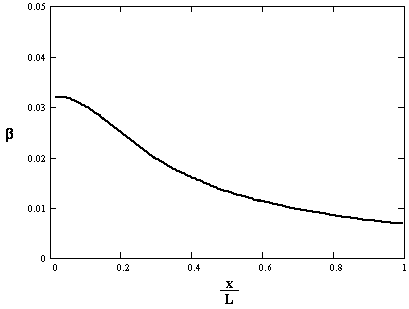Fig. 4 Axial Distribution of the Free-Flow Surface Area Fraction

14 of 18Fig. 5 Predicted Axial Pressure Distribution

15 of 18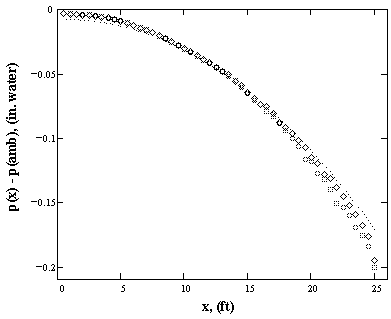Fig. 6 Comparison of Measured and Predicted Pressure Distributions

16 of 18

#### 5. CONCLUDING REMARKS

This work was undertaken to develop a method for increasing the utilization of attic-

collected solar energy. The basic idea is to draw air from all parts of the attic space into the inlet

face of a heat exchanger which facilitates the utilization of the solar-heated attic air. In the

practical realization of this idea, a flexible conduit attached to the inlet face of the exchanger is

deployed throughout the attic space. The wall of the conduit is made permeable to enable air to

be drawn into it from all attic neighborhoods along its length. The permeability is adjusted so

that air is ingested uniformly along the entire length of the conduit, and the far end of the conduit

is capped.

An analytical model was developed to determine the axial variation of the conduit-wall

permeability needed to achieve the uniform ingestion condition. The model yielded closed-form,

algebraic solutions for both the axial distributions of the permeability and the static pressure.

An experimental facility was fabricated to test the validity of the model and its results. A

conduit was made permeable in accordance with the prescription provided by the analysis, and

axial pressure distributions were measured. The measured pressure distributions were found to

be in very good agreement with that predicted by the analysis. This level of agreement validated

the model and supports its further use as a design tool to enhance the utilization of attic-collected

solar energy.

17 of 18

#### NOMENCLATURE

D Diameter of conduit Friction factor Length of conduit Rate of ingestion per unit length Air flow rate at any x Air flow rate at conduit exit Dimensionless pressure, equation (22) Ambient pressure in attic Static pressure at x Static pressure at conduit exit Reynolds number Wall shear stress Dimensionless axial coordinate, x/L Axial coordinate Air velocity Air velocity passing through conduit wall; Axial velocity at x Fraction of wall surface area that is open to airflow Air viscosity Air density

REFERENCES
 (1) Duffie, J. A. and Beckman, W. A., Solar Engineering of Thermal Processes, John Wiley & Sons, New York, 1991. (2) Kreith, F and Kreider, J. F., Principles of Solar Engineering, McGraw-Hill, New York, 1978. (3) PCS1 SolarAttic Pool Heater Manual, SolarAttic, Elk River, Minnesota, 1991.

18 of 18